Next      Up      Previous      Contents

# Geometry editing

Geometry editor is used to measure and change the geometric parameters of the model. It can be invoked from the Analyze >Geometry or Edit > Geometry menus or by pressing the toolbar button.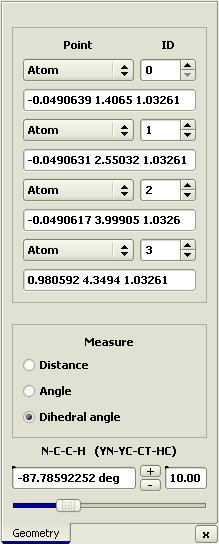Measurements can be made between 2, 3, or 4 points in space. Accordingly, the distance, angle, and dihedral angle are measured. Atomic centers, molecular centers-of-mass, or arbitrary points specified by Cartesian coordinates can be used as spatial points.

A measurement between several atoms can be performed by mouse picking them or by specifying their numbers. Note that the numbering starts from zero.

The logic of the editor actions depends on the points selected. If two atoms connected by a chemical bond were selected the molecule is divided into two parts by this bond. The part associated with the first atom remains static, while the other part is shifted to correspond to the specified value. If two atoms not bound then the editor shifts all atoms in the molecule. Thus, a molecule can be shifted as a whole along any line connecting two atoms. In addition, any point specified by Cartesian coordinates or an atom in a different molecule can be selected as the first point.

Angles or dihedral angles are changed in a similar way. If the angle between bonds in the same molecule can be changed by rotating a part of the molecule, such rotation is performed; otherwise, the molecule is rotated as a whole.

Let us exemplify working in the editor by a practical task to arrange the molecules of water and methane in a configuration corresponding to a local energy minimum. In this case, the oxygen atom of water is opposite to the hydrogen atom in methane at a distance of 3.76 Å from the carbon atom. The hydrogen atoms of water point away from the methane molecule.

 Open file of a methane molecule (CH4.hin)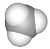add a water molecule: File > Add > Water.hin + OK button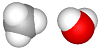set the distance between the C and O atoms to 3.76 Å (atoms 0–5)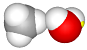set the C–H–O angle to 180° (atoms 0–1–5)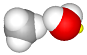set the C–O–mc angle to 180° (mc is water center-of-mass (mol 1))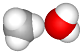set the H–C–O–H dihedral angle to 0° (atoms 2–0–5–6)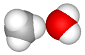since the distance between the oxygen and carbon slightly changed after the manipulations with the center-of-mass, reset it to 3.76 Å (atoms 0–5)

This yields the desired model: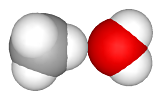The torsion angles can be also changed outside of the Geometry editor by editing the mlm file. This is done by specifying the type and value of the altered torsion angle in the following tables.

 @Table Torsion_types str str str str str str Type Fine1 Fine2 Fine3 Fine4 Comment CH=CH HC=1 CH=1 CH=2 HC=2

Here, a torsion angle type CH=CH is specified. It is defined as a torsion angle between the atoms with the Fine types HC=1, CH=1, CH=2, and HC=2. After angle types have been specified, their values can be set. For instance, the table

 @Table Torsions str double str str Type Value Res Comment CH=CH 0 1

sets the torsion angle of 0° about the double bond, i.e., a cis-conformation.

Next      Up      Previous      Contents